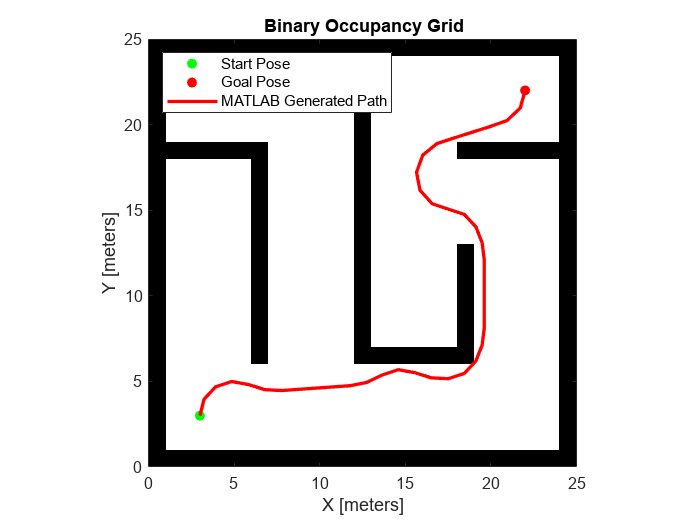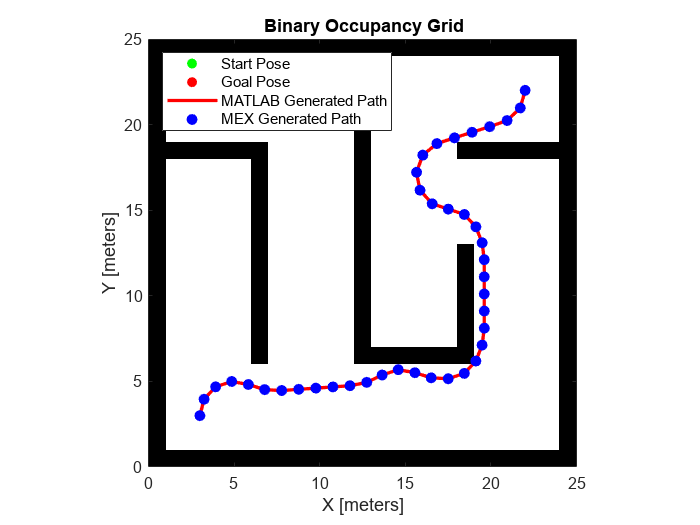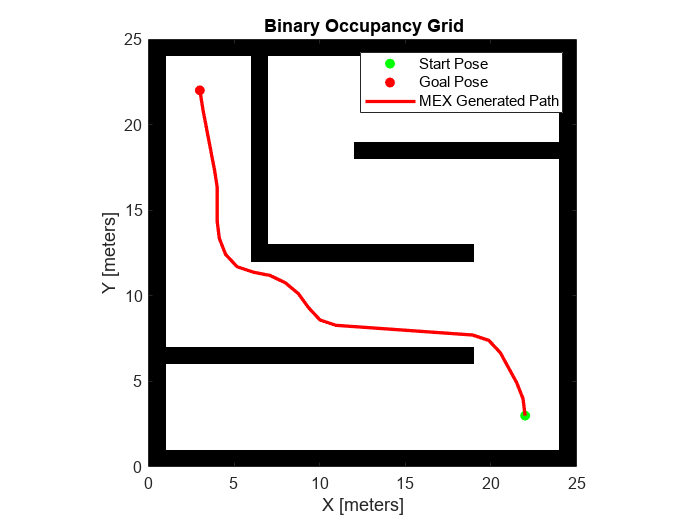Generate Code for Path Planning Using Hybrid A Star

This example shows how to perform code generation to plan a collision-free path for a vehicle through a map using the Hybrid A* algorithm. After you verify the algorithm in MATLAB®, use the generated MEX file in the algorithm to visualize the planned path.

Write Algorithm to Plan Path

Create a function, codegenPathPlanner, that uses a plannerHybridAStar object to plan a path from the start pose to the goal pose in the map.

function path = codegenPathPlanner(map,startPose,goalPose)
% Copyright 2021 The MathWorks, Inc.
%#codegen
% Create a state space object
stateSpace = stateSpaceSE2;

% Create a state validator object
validator = validatorOccupancyMap(stateSpace);

% Create a binary occupancy map and assign the map to the state
% validator object.
validator.Map = binaryOccupancyMap(map);

% Set the validation distance for the validator
validator.ValidationDistance = 0.01;

% Assign the state validator object to the plannerHybridAStar object
planner = plannerHybridAStar(validator);

% Compute a path for the given start and goal poses
pathObj = plan(planner,startPose,goalPose);

% Extract the path poses from the path object
path = pathObj.States;
end

This function acts as a wrapper for a standard Hybrid A* path planning call. It accepts standard inputs and returns a collision-free path as an array. Because you cannot use a handle object as an input or output of a function that is supported for code generation, create the planner object inside the function. Save the codegenPathPlanner function in your current folder.

Verify Path Planning Algorithm in MATLAB

Verify the path planning algorithm in MATLAB before generating code.

Load a map into the workspace.

Create a state space object.

stateSpace = stateSpaceSE2;

Create a state validator object.

stateValidator = validatorOccupancyMap(stateSpace);

Create a binary occupancy map and assign the map to the state validator object.

stateValidator.Map = binaryOccupancyMap(map);

Set the validation distance for the validator.

stateValidator.ValidationDistance = 0.01;

Initialize the plannerHybridAStar object with the state validator object.

planner = plannerHybridAStar(stateValidator);

Define start and goal poses as [x y theta] vectors. x and y specify the position in meters, and theta specifies the orientation angle in radians.

startPose = [5 5 pi/2];
goalPose = [22 4 0];

Plan a path from the start pose to the goal pose.

plan(planner,startPose,goalPose);

Visualize the path using the show function, and hide the expansion tree.

show(planner,Tree="off")
hold onGenerate Code for Path Planning Algorithm

You can use either the codegen (MATLAB Coder) function or the MATLAB Coder (MATLAB Coder) app to generate code. For this example, generate a MEX file by calling codegen at the MATLAB command line. Specify sample input arguments for each input to the function using the -args option and func_inputs input argument.

Call the codegen function and specify the input arguments in a cell array. This function creates a separate codegenPathPlanner_mex function to use. You can also produce C code by using the options input argument. This step can take some time.

codegen codegenPathPlanner -args {map,startPose,goalPose}
Code generation successful.

Verify Results Using Generated MEX Function

Plan the path by calling the MEX version of the path planning algorithm for the specified start pose, and goal pose, and map.

path = codegenPathPlanner_mex(map,startPose,goalPose);

Visualize the path computed by the MEX version of the path planning algorithm.

scatter(path(:,1),path(:,2),...
Marker="o",...
MarkerFaceColor="b",...
MarkerEdgeColor="b")
legend("MATLAB Generated Path","Start Pose","Goal Pose","MEX Generated Path")
hold offCheck Performance of Generated Code

Compare the execution time of the generated MEX function to the execution time of your original function by using timeit.

time = timeit(@() codegenPathPlanner(map,startPose,goalPose))
time =
0.1317215226

mexTime = timeit(@() codegenPathPlanner_mex(map,startPose,goalPose))
mexTime =
0.0208781226

time/mexTime
ans =
6.30906931258273

In this example, the MEX function runs more than five times faster. Results may vary for your system.

Plan Path in New Map Using Generated MEX Function

Plan a path for a new start and goal poses in a new map. The size of the new map must be the same as the map used to generate the MEX function.

startPoseNew = [10 8 pi];
goalPoseNew = [5 22 0];
pathNew = codegenPathPlanner_mex(mapNew,startPoseNew,goalPoseNew);

Visualize the new path computed by the MEX function.

show(binaryOccupancyMap(mapNew))
hold on
scatter(pathNew(:,1),pathNew(:,2),...
Marker="o",...
MarkerFaceColor="b",...
MarkerEdgeColor="b")